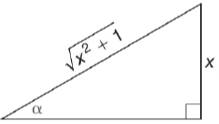Chapter 11.3, Problem 9E### Elementary Geometry for College St...

6th Edition
Daniel C. Alexander + 1 other
ISBN: 9781285195698

#### Solutions

Chapter
Section### Elementary Geometry for College St...

6th Edition
Daniel C. Alexander + 1 other
ISBN: 9781285195698
Textbook Problem
1 views

# In Exercises 5 to 10, find the value (or expression) for each of the six trigonometric ratios of angle α . Use the Pythagorean Theorem as needed.To determine

To find:

The value for each of the six trigonometric ratios of angle α.

Explanation

Given:

The given figure is

Definition used:

The six trigonometric ratios:

Calculation:

Let the unknown side in the triangle be a.

(x2+1)2=x2+(a)2x2+1=x2+a21=a21=a21=a

Thus we have

From the figure, opposite=x, adjacent=1, hypotenuse=x2+1

### Still sussing out bartleby?

Check out a sample textbook solution.

See a sample solution

#### The Solution to Your Study Problems

Bartleby provides explanations to thousands of textbook problems written by our experts, many with advanced degrees!

Get Started

#### Find more solutions based on key concepts